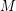# Samal, Robert

## Star chromatic index of complete graphs ★★

Author(s): Dvorak; Mohar; Samal

Conjecture   Is it possible to color edges of the complete graphusingcolors, so that the coloring is proper and no 4-cycle and no 4-edge path is using only two colors?

Equivalently: is the star chromatic index oflinear in?

Keywords: complete graph; edge coloring; star coloring

## Star chromatic index of cubic graphs ★★

Author(s): Dvorak; Mohar; Samal

The star chromatic indexof a graphis the minimum number of colors needed to properly color the edges of the graph so that no path or cycle of length four is bi-colored.

Question   Is it true that for every (sub)cubic graph, we have?

Keywords: edge coloring; star coloring

## Weak pentagon problem ★★

Author(s): Samal

Conjecture   Ifis a cubic graph not containing a triangle, then it is possible to color the edges ofby five colors, so that the complement of every color class is a bipartite graph.

## Drawing disconnected graphs on surfaces ★★

Author(s): DeVos; Mohar; Samal

Conjecture   Letbe the disjoint union of the graphsandand letbe a surface. Is it true that every optimal drawing ofonhas the property thatandare disjoint?

Keywords: crossing number; surface

## Cores of Cayley graphs ★★

Author(s): Samal

Conjecture   Letbe an abelian group. Is the core of a Cayley graph (on some power of) a Cayley graph (on some power of)?

Keywords: Cayley graph; core